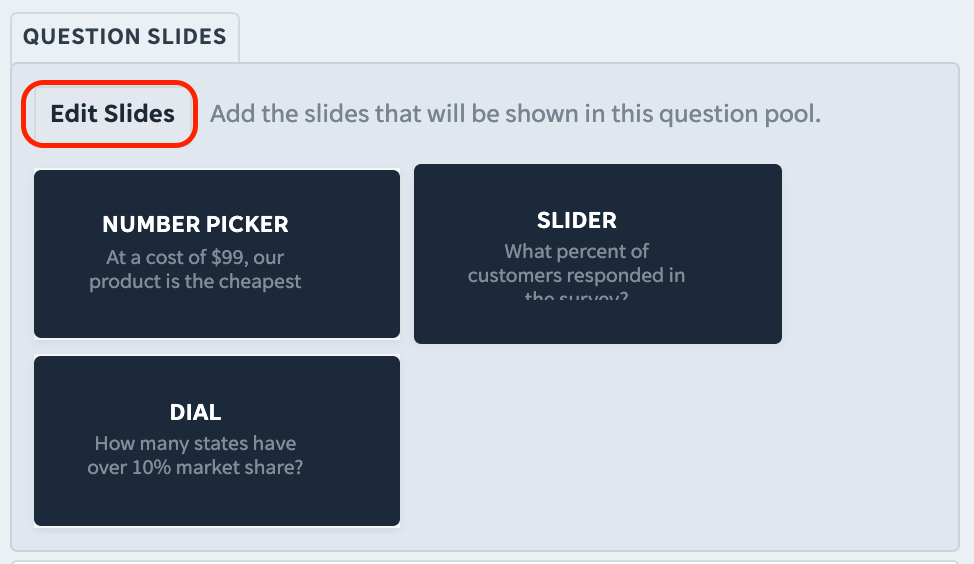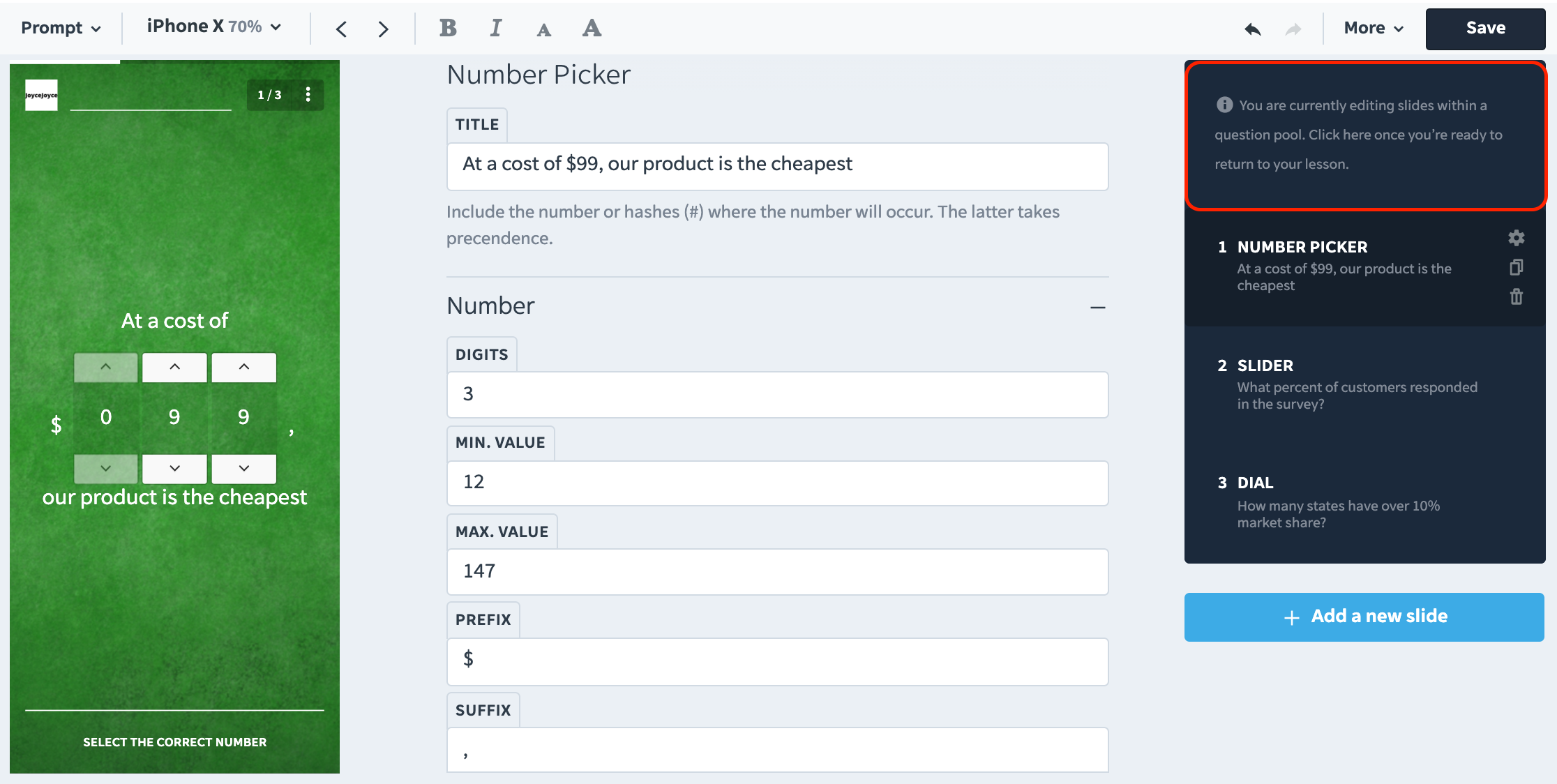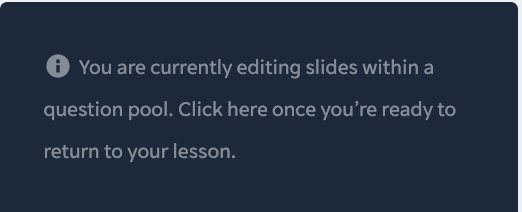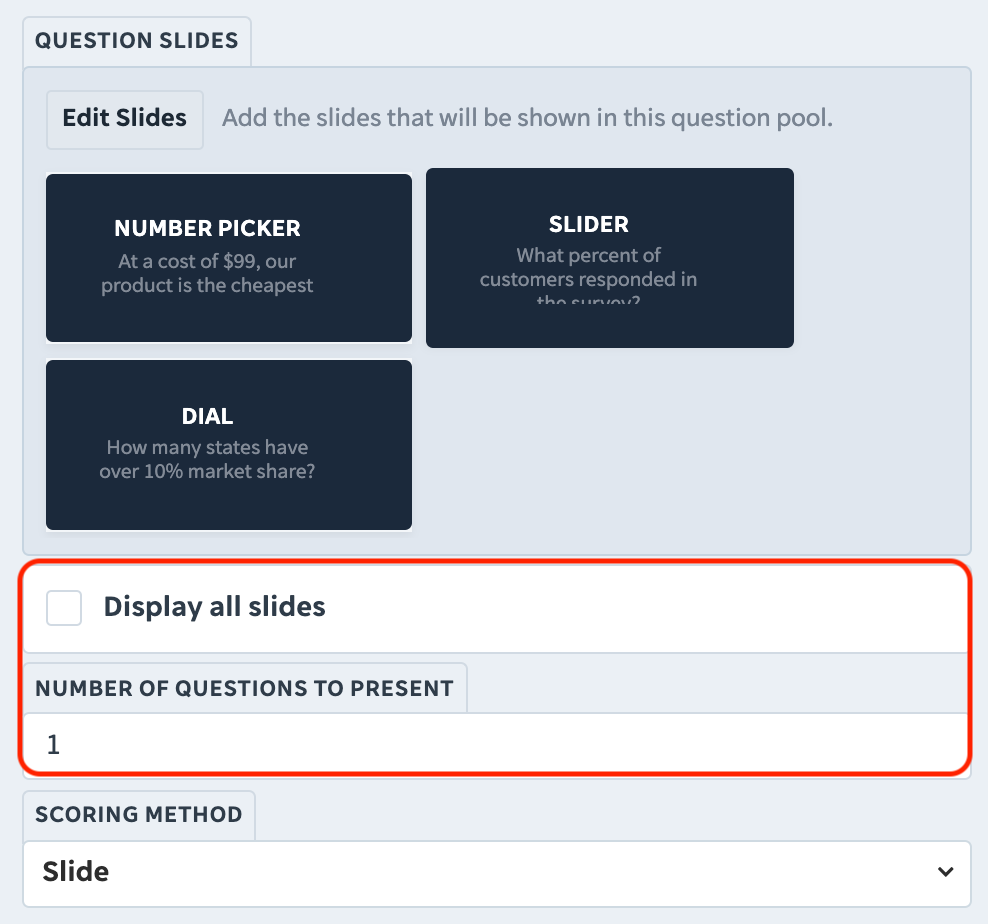## The Question Pool Advanced Template lets authors ask a variety of questions using different quiz templates, while only using one lesson slide. Here’s how it works.

Question Pool is an advanced template that keeps learners engaged through a variety of quiz templates given in rapid succession.

#### How it works

The Question Pool template lets authors engage learners by giving them a chain of various quiz templates e.g. Concepts, Multiple Choice, Numbers, Relationships, all within just one lesson slide.

Learners have to answer an ordered or randomized selected amount of questions from a larger 'pool' of questions.

#### What it’s for

It's great for creating “Quiz Lessons”. These are EdApp Lessons composed entirely of questions, and are meant to be taken after completing prior courses.

### Configuring a Question Pool Advanced Template

After selecting Question Pool from the Advanced template category, it opens up to a default of three quiz templates; namely, the Number Picker, Slider, and the Dial.

Step 1: Click on ‘Edit Slides’ to select only the quiz templates you would like to include.Step 2: You will be brought to the Question Pool’s editing page. It looks similar to the regular content authoring page, except for the message at the top of the lesson slide column:While in here, you can add and rearrange quiz templates within the question pool, as you normally would. Rearranging quiz templates here is especially important if you want the questions to be asked in a specific order.

Note:

• All of the quiz slides you include in this editing space are contained within the question pool slide only
• You can add as many quiz templates as you want
• You cannot include Content, Games, Survey, and other Advanced templates inside a Question Pool (these options will be grayed out within the question pool editing page)

Step 3: Once you’re done editing the quiz templates within the question pool, simply click on the message at the top of the slide column, and you’ll be brought back to the standard lesson editing page.If you want the quiz templates within your question pool to be asked in order, tick the ‘Display all slides’ box.

If you want the questions to be asked in a random order, untick the ‘Display all slides’ box, and instead specify the NUMBER OF QUESTIONS TO PRESENT found just below the tick box.

### Scoring Method

The last thing that you need to do, is to set the Scoring Method. There are five different options for scoring, so you should pick the one that fits your testing preference best.

#### Method 1: Slide Scoring

Score = (Number of Correct Slides ÷ Number of Total Slides) × 100Example:

In a multiple-choice slide, the learner needs to select all correct answers only in order to get a score of 1.

If the learner happens to select any incorrect answers in addition to selecting all of the correct answers, they still get a score of 0.

With the Slide scoring method, the total score for the Question Pool is computed based on the number of slides the learner answered correctly.

Score = (Number of Correct Answers ÷ Number of Total Correct Answers) × 100Example:

In the example above, there are a total of three correct answers (Blue from Blues Clues, Scooby-Doo, and Snoopy)

However, the learner only got two answers correct (Blue from Blues Clues, and Scooby-Doo)

The formula then computes the score as follows:
(2 ÷ 3) × 100 = 67%

The learner gets a total score of 67%

With the Answer scoring method, the total score for the Question Pool is computed based on the total number of correct answers, not necessarily the total number of correct slides.

#### Method 3: Slide Negative Scoring

Score = (Number of Correct Slides - Number of Incorrect Slides) ÷ Total Number of Slides × 100

This scoring method works almost the same way as Slide, except that it penalizes learners if they answer a slide incorrectly.

Example:

There’s a total of 3 slides in the question pool. If slides 1 and 2 are answered correctly (+2), but slide 3 is answered incorrectly (-1), the result is a net score of 1.

(1 ÷ 3) × 100 = 33% total score

If more slides are answered incorrectly than correctly, the learner cannot receive a score less than 0.

#### Method 4: Answer Negative Marking

Score = (Number of Correct Answers - Number of Incorrect Answers) ÷ Total Number of Answers × 100

Essentially a combination of the Answer and the Slide Negative scoring methods, this mode rewards the learner for each individually correct answer, but also penalizes them for each individually incorrect answer.
In this scoring method, a correct answer can be defined in two ways:
- correct answer is selected (+1); or
- an incorrect answer is not selected (+1)

An incorrect answer is also defined in two ways:
- an incorrect answer is selected (-1); or
- a correct answer is not selected (-1)

Example:“Stuart Little” is correct because he is not a dog, and the learner did not select him (+1)
“Blue from Blues Clues” is correct because he is a dog, and the learner selected him (+1)
“Scooby-Doo” is correct because he is a dog, and the learner selected him (+1)

“Simba” is incorrect because he is not a dog, but the learner selected him (-1)
“Snoopy” is incorrect because he is a dog, but the learner did not select him (-1)

So out of the 5 possible answers, the score is computed as such:
(1 + 1 + 1 - 1 - 1) ÷ 5 × 100 = 20%

The total score for the learner is 20%

If the learner gets more incorrect answers than correct answers, the learner cannot receive a score less than 0.

#### Method 5: Answer Negative Marking II

This scoring method uses the same formula as Answer Negative Marking.

The only difference is, if the learner attempts to either answer nothing or answer everything, they get a score of zero from the slide.

Note that this scoring method relies on quiz slides to not be set up in a way that ALL of the choices are correct answers.

### Summary

The Question Pool advanced template is a great way to test your learner’s memory. It also keeps them engaged by presenting a variety of quiz slides to answer so that assessments never get too dull or predictable.